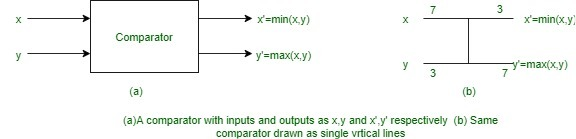# Comparison Networks

Comparison Networks are a type of sorting networks which always sort their inputs. Wires and comparator comprise comparison network. A Comparator is a device which has two inputs (x, y) and outputs (x’, y’). It performs the following function:

```x' = min(x, y),
y' = max(x, y) ```Comparators are conventionally drawn as single vertical lines where input is on the left and output on right. Smaller input values are put on top output while larger input values are put on bottom output. thus comparator can be thought of as sorting its two inputs. It is assumed that each comparator operates in O(1) time i.e time between appearance of input values and production of output is constant.

The second component of comparison networks i.e. wires performs the function of transmitting a value from one place to another. These are either network input wires or network output wires. Wires are capable of connecting input of a comparator to output of other.

Output is produced by comparator only when it has both of its input values available. Now, assuming that each comparator takes unit time “running time” can be defined. Running time is time taken by output wires to receive values after input wires have received their values. Formally is defined as follows. An input wire of comparison network has depth 0. Now, if d1 and d2 are depths of two input wires, then the depth of output wires is max(d1, d2) + 1. Depth of a comparator is defined to be the depth of its output wires.

Properties:

• Graph is required to be acyclic.
• Output is produced only when input is available.
• Comparators process in parallel if input is available.
• Sorting network is a comparison network where the output is sorted.
• Not all comparison networks are sorting networks.
• A comparison network is like a procedure which specifies how comparisons are to occur.

Theorems:

1. If a comparison network with n inputs sorts all 2n possible sequences of 0’s and 1’s correctly, then it sorts all sequences of arbitrary numbers correctly.
2. If a comparison network transforms the input a = ha1, a2, . . ., ani into the output b = hb1, b2, . . ., bni, then for any monotonically increasing function f, the network transforms
```f(a) = hf(a1), f(a2), . . ., f(an)i
into
f(b) = hf(b1), f(b2), . . ., f(bn)i ```

Don’t stop now and take your learning to the next level. Learn all the important concepts of Data Structures and Algorithms with the help of the most trusted course: DSA Self Paced. Become industry ready at a student-friendly price.

My Personal Notes arrow_drop_upCheck out this Author's contributed articles.

If you like GeeksforGeeks and would like to contribute, you can also write an article using contribute.geeksforgeeks.org or mail your article to contribute@geeksforgeeks.org. See your article appearing on the GeeksforGeeks main page and help other Geeks.

Please Improve this article if you find anything incorrect by clicking on the "Improve Article" button below.

Article Tags :

Be the First to upvote.

Please write to us at contribute@geeksforgeeks.org to report any issue with the above content.